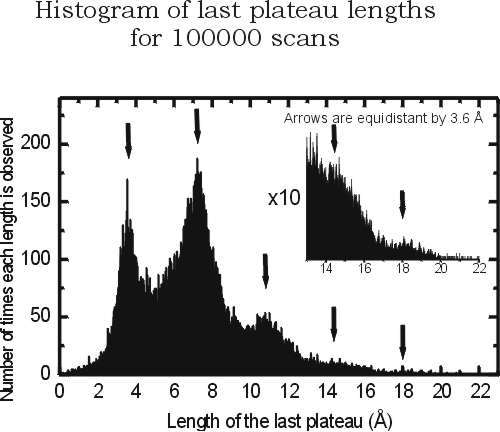# Draw 2in1 histogram

Hello,

I would like to have a histogram containing another histogram in it, e.g. there is a histogram and there is more one which is a scaled version of the original one. Something like my attachment Pic. (see below).

Could you suggest me a possible solution in root? Thanks in advance!see example below

Rene

```void spad() { TCanvas *c1 = new TCanvas; TH1F *h = new TH1F("h","test",100,-3,7); h->FillRandom("gaus",10000); h->Draw(); TPad *smallpad = new TPad("smallpad","",0.5,0.4,0.85,0.78); smallpad->Draw(); smallpad->cd(); TH1F *h2 = (TH1F*)h->Clone("h 2"); h2->GetXaxis()->SetRange(10,47); h2->Draw(); c1->cd(); }```

Could you give me some hint to execute this example?

I tried to run this in the following way, but I get error.

``````root  .x example_hist.C
Error: Function example_hist() is not defined in current scope  :0:
*** Interpreter error recovered ***
root  ``````

Sorry, but I am a newbie in root.

name the script spad.C and do

``` root > .x spad.C ```or if your file is already named example_hist.C, do

``` root > .L example_hist.C root > spad() ```
see the first pages of teh Users Guide

Rene